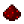# Turtle.forward

Jump to: navigation, searchFunction turtle.forward Attempts to move the turtle forward. Syntax turtle.forward() Returns boolean whether the turtle succeeded in moving forward Part of ComputerCraft API turtle

## ExamplesExample Tries to move the turtle forward and outputs whether it worked or not. Code ```if turtle.forward() then print ("Turtle moved forward.") else print ("Turtle didn't move forward.") end ``` Output Turtle moved forward. or Turtle didn't move forward.Example Tries to move the turtle forward a number of times. The times to move forward is specified by the user. Code ```local distance = ... -- gets the first argument from the user if distance ~= nil then distance = tonumber (distance) -- make it a number for i = 1, distance do turtle.forward() end else print ("Did not specify distance.") end ``` Output Either the turtle moving the specified amount of times or missing parameter.Example Digs until the turtle can move forward. Code ```while not turtle.forward() do turtle.dig() end ``` Output The turtle moves forward.